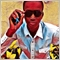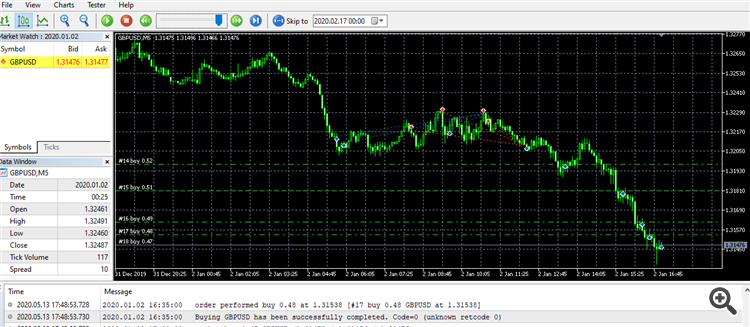# Martingale Lot Increase On Next Signal3644

Hello.. i cant figure out whats wrong with my code..

the lot decreases on next signal instead to increase

```input string MartinGale = "===< MartinGale Settings >===";
input double          Risk=1.0;
input double          Multi=0.5;
```
```double          MinLot,MaxLot,Contract,lot;
int             DealNumber;
long            Leverage;
```
```int OnInit()
{
Leverage=AccountInfo.Leverage();
MinLot=SymbolInfo.LotsMin();
MaxLot=SymbolInfo.LotsMax();
Contract=SymbolInfo.ContractSize();
DealNumber=0;
return(0);
}
void OnTick()
{
{
DealNumber++;
Lot=AccountInfoDouble(ACCOUNT_FREEMARGIN)*Risk/100*Leverage/ContractSize;
if(DealNumber>1)Lot=Lot*Multi*(DealNumber-1);Lot=NormalizeDouble(Lot,2);
if(Lot<MinLot)Lot=MinLot;if(Lot>MaxLot)Lot=MaxLot;

MqlTick last_tick;

DealNumber--;

}
}

}
```

if i change this it will increase lot on every new signal.. but will continue to increase if Total Orders is = 0

`DealNumber++;`

with this.. it will restart if Total orders is =0

`DealNumber--;`

Ill really appreciate mod to my code37084

Abubakar Saidu:

Hello.. i cant figure out whats wrong with my code..

the lot decreases on next signal instead to increase

if i change this it will increase lot on every new signal.. but will continue to increase if Total Orders is = 0

with this.. it will restart if Total orders is =0

Ill really appreciate mod to my code

Hello,

try something this....

```input string MartinGale = "===< MartinGale Settings >===";
input double          Risk=1.0;
input double          Multi=0.5;
double          MinLot,MaxLot,Contract,lot;
int             DealNumber;
long            Leverage;

//+------------------------------------------------------------------+
//|                                                                  |
//+------------------------------------------------------------------+
int OnInit()
{
Leverage=AccountInfo.Leverage();
MinLot=SymbolInfo.LotsMin();
MaxLot=SymbolInfo.LotsMax();
Contract=SymbolInfo.ContractSize();
DealNumber=0;
return(0);
}

//+------------------------------------------------------------------+
//|                                                                  |
//+------------------------------------------------------------------+
void OnTick()
{
{
if(OrdersTotal()>0)
DealNumber++;
else
DealNumber=0;

int ticket=-1;

Lot=AccountInfoDouble(ACCOUNT_FREEMARGIN)*Risk/100*Leverage/ContractSize;

if(DealNumber>1)
Lot=Lot*Multi*(DealNumber-1);
Lot=NormalizeDouble(Lot,2);
if(Lot<MinLot)
Lot=MinLot;
if(Lot>MaxLot)
Lot=MaxLot;

MqlTick last_tick;

}
}```3644

i tried it and it was not increasing the lot size on new signal..3644

Tried this also lot not increasing on new signals

```input string MartinGale = "===< MartinGale Settings >===";
input double          Risk=1.0;
input double          Multi=0.5;
double          MinLot,MaxLot,Contract,lot;
int             DealNumber;
long            Leverage;
input double          LotIncrease      =0.9;
input double         StartLot=0.5;

//+------------------------------------------------------------------+
//|                                                                  |
//+------------------------------------------------------------------+
int OnInit()
{
Leverage=AccountInfo.Leverage();
MinLot=SymbolInfo.LotsMin();
MaxLot=SymbolInfo.LotsMax();
Contract=SymbolInfo.ContractSize();
DealNumber=0;
return(0);
}

//+------------------------------------------------------------------+
//|                                                                  |
//+------------------------------------------------------------------+
void OnTick()
{
{
if(OrdersTotal()>0)
DealNumber++;
else
DealNumber=0;

int ticket=-1;

Lot=AccountInfoDouble(ACCOUNT_FREEMARGIN)*Risk/100*Leverage/ContractSize;

if(DealNumber>1)
Lot=Lot*Multi*(DealNumber-1);
Lot=NormalizeDouble(Lot,2);
if(Lot<MinLot)
Lot=MinLot;
if(Lot>MaxLot)
Lot=MaxLot;

MqlTick last_tick;

}
}

double mart()
{
double fb1,fb2;
double lots=0;
fb1= StartLot;
fb2=fb1*LotIncrease;
fb1= StartLot;
fb1=fb1*LotIncrease;

return(fb1);
}
{
for(int i=0;i<OrdersTotal();i++)
{
int sb=OrderSelect(i);
if(PositionGetInteger(POSITION_TYPE)==0 && PositionGetString(POSITION_SYMBOL)==Symbol() && PositionGetInteger(POSITION_MAGIC)==MagicNumber)
}
}

double fbo()
{
ENUM_POSITION_TYPE type=(ENUM_POSITION_TYPE)PositionGetInteger(POSITION_TYPE);    // type of the position
double openprice = PositionGetDouble(POSITION_PRICE_OPEN);
double z=0,zz=0;
for(int i=OrdersTotal();i>=0;i--)
{
int a=OrderSelect(i);
{
z=openprice;
if(z<zz || zz==0)zz=z;
}
}
return zz;
}
```

Oooohh..56

since youre counting buy orders, who not just calculate the lostize of the next buy order in that function?

each time through the loop your global buy_lotsize variable gets increased accordingly.

Although martingale is a fools errand..... Ive seen a proof that shows that probability of doubling your account is always exactly equal to the probability of a single winning outcome, and the probability of zeroing your account is equal to the probability of a single losing outcome....3644

i want to try something with martingale..

can you modify the wrong part of my code!!37084

Abubakar Saidu:

i want to try something with martingale..

can you modify the wrong part of my code!!

You code not working.21914

Martingale, guaranteed to blow your account eventually. If it's not profitable without, it is definitely not profitable with.
Martingale vs. Non Martingale (Simplified RoR vs Profit and the Illusions) - MQL5 programming forum 2015.02.11

Why it won't work: Calculate Loss from Lot Pips - MQL5 programming forum 2017.07.113644

Nikolaos Pantzos:

You code not working.

hi.. thanks for your responses .. i have tried many possible ways .. still not working

can you highlight the wrong thing in my code3644

William Roeder:
Martingale, guaranteed to blow your account eventually. If it's not profitable without, it is definitely not profitable with.
Martingale vs. Non Martingale (Simplified RoR vs Profit and the Illusions) - MQL5 programming forum 2015.02.11

Why it won't work: Calculate Loss from Lot Pips - MQL5 programming forum 2017.07.11

Hi.. yes i know i just want to try something with it!!!56

Abubakar Saidu:
Hi.. yes i know i just want to try something with it!!!
```//--// some pseudocode used in this example: do not copypasta: fix it.
double lotsize(void){
if (OrdersTotal() == 0) return(StartLot); //does this even need a comment??
// OrdersTotal must be >0 to get to this line
double ret=StartLot; // ret is just short for "return value"
for(int i=OrdersTotal();i!=0;i--){ // you may as well count down as up, often its easier and comparing to zero usually takes less clock cycles
if(get the right order type) ret *= LotIncrease; //since you have a nice exponential lotsize formula, just use it
}
// ret should now be the next lotsize in the sequence.
return(ret); //ummm??
}```